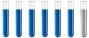## Molarity, weight %, Molality and other variables of solution

Chemistry and homework help forum.

Organic Chemistry, Analytical Chemistry, Biochemistry, Physical Chemistry, Computational Chemistry, Theoretical Chemistry, High School Chemistry, Colledge Chemistry and University Chemistry Forum.

Share your chemistry ideas, discuss chemical problems, ask for help with scientific chemistry questions, inspire others by your chemistry vision!

Please feel free to start a scientific chemistry discussion here!

Discuss chemistry homework problems with experts!

Ask for help with chemical questions and help others with your chemistry knowledge!

Moderators: expert, ChenBeier, Xen

Dhamnekar Winod
Sr. Staff MemberPosts: 156
Joined: Sat Nov 21, 2020 10:14 am
Location: Mumbai[Bombay],Maharashtra State,India

### Molarity, weight %, Molality and other variables of solution

A solution is prepared by 10 g of solid para-dichlorobenzene (C6H4Cl2) in enough carbon tetrachloride to make a total of 350 mL of solution. The density of the resulting solution is 1.60 g/mL .

(a) Compute the molarity of the solution.

(b) Compute the weight % para-dichlorobenzene of the solution.

(c)Compute the molality of the solution.

(d) Compute the mole fraction of the solute.

(e) At 23℃ the vapor pressure of CCl4 is 100 mm. Compute the vapor pressure of the solution at this temperature.

(f) When a different solution of C6H4Cl2 is prepared, it is found to have a boiling point of 78.6℃. Given that $$K_b$$ = 5.0 ℃-kg/mol and the boiling point of pure CCl4 is 76.6℃, compute how many grams of solute are present in 100 grams of CCl4.

I am working on this question and all of its sub-questions.
Any science consists of the following process. 1) See 2)Hear 3)Smell,if needed 4)Taste, if needed 5)Think 6)Understand 7)Inference 8)Take decision [Believe or disbelieve, useful or useless, true or false, cause or effect, any other criteria]
ChenBeier
Distinguished MemberPosts: 776
Joined: Wed Sep 27, 2017 7:25 am
Location: Berlin, Germany

### Re: Molarity, weight %, Molality and other variables of solution

a) 0,194 M
b) 1,79% w.w
c) 0,12 mmol/kg
d) 0,019
The Rest its your job.
Dhamnekar Winod
Sr. Staff MemberPosts: 156
Joined: Sat Nov 21, 2020 10:14 am
Location: Mumbai[Bombay],Maharashtra State,India

### Re: Molarity, weight %, Molality and other variables of solution

Thanks for your correct answers to questions (a), (b), (c) and(d).

My Computation work for answers to question (e) and (f).

My answer to (e)$$P_{CCl_4}=0.981 \times 100 mm=98.1 mm, P_{CCl_4}=$$ Vapor pressure of solution

My answer to (f) $$\Delta T_{bp}=K_{bp}\times m; m= \frac{\Delta T_{bp}}{K_b}=\frac{78.6^\circ C-76.6^\circ C}{5.02^\circ C-kg/mol}=0.40$$molal

Moles of C6H4Cl2= 0.40 kg/mol × 0.100 kg = 0.040 mol C6H4Cl2

Grams of C6H4Cl2 = 0.040 mol C6H4Cl2 × 147 g/mol =5.88 g C6H4Cl2
Any science consists of the following process. 1) See 2)Hear 3)Smell,if needed 4)Taste, if needed 5)Think 6)Understand 7)Inference 8)Take decision [Believe or disbelieve, useful or useless, true or false, cause or effect, any other criteria]
ChenBeier
Distinguished MemberPosts: 776
Joined: Wed Sep 27, 2017 7:25 am
Location: Berlin, Germany

Ok